# Test 4 Review 2 Hamburgers cost 2 each

• Slides: 41Test 4 Review2. Hamburgers cost \$2 each and pizzas cost \$6 each. If the number of hamburgers was 2 more than twice the number of pizzas and if a total of \$84 was spent, how many hamburgers and how many pizzas were purchased.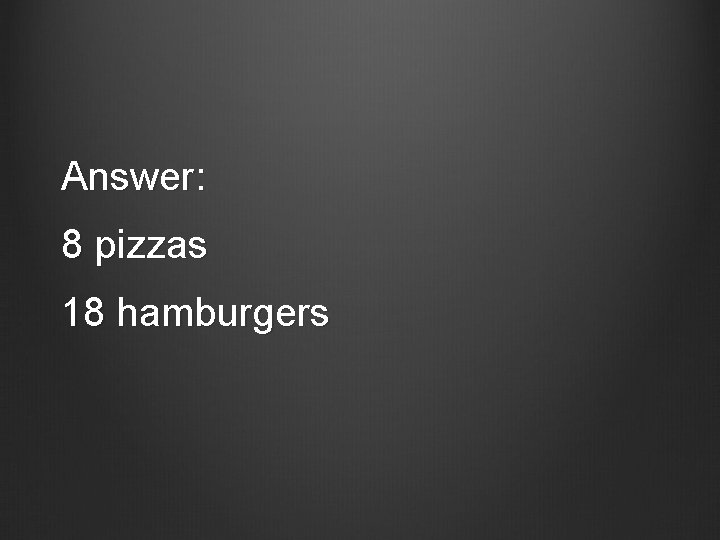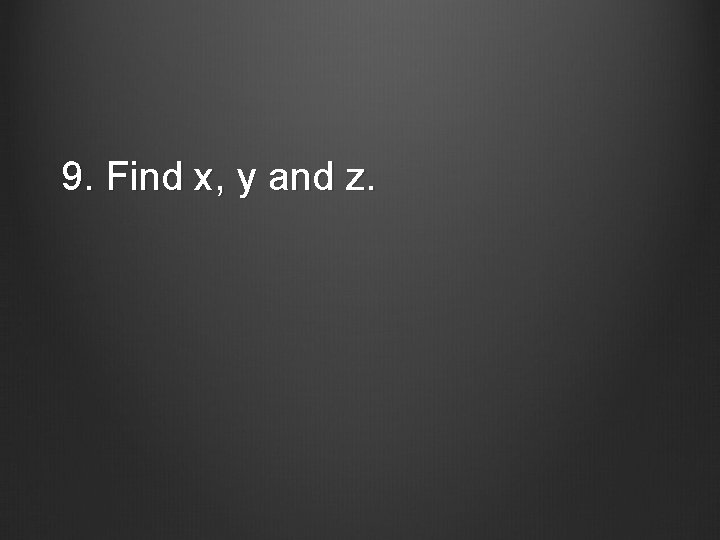9. Find x, y and z.Answer: Z = 170 X = 120 Y = 105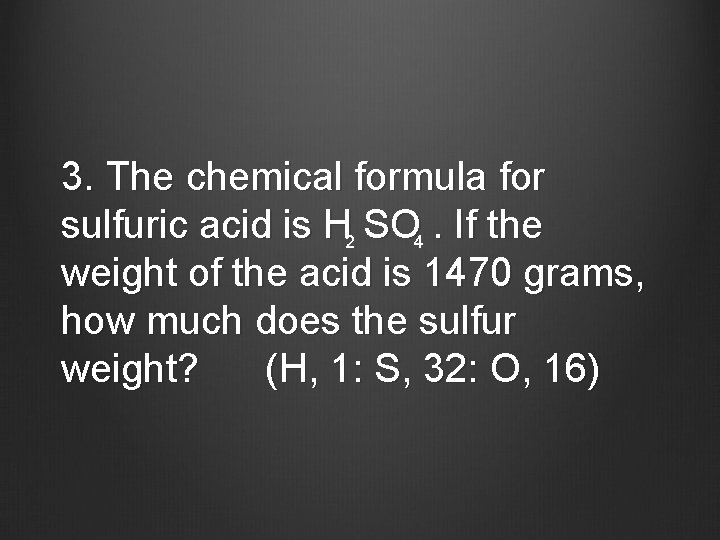3. The chemical formula for sulfuric acid is H 2 SO 4. If the weight of the acid is 1470 grams, how much does the sulfur weight? (H, 1: S, 32: O, 16)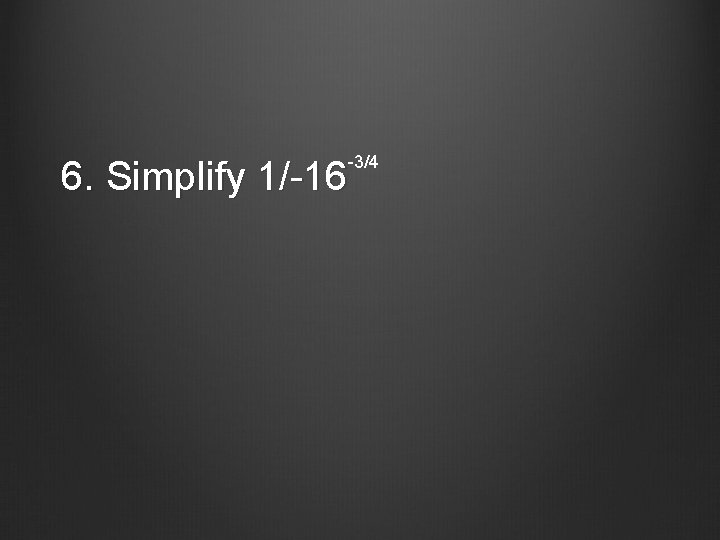-3/4 6. Simplify 1/-16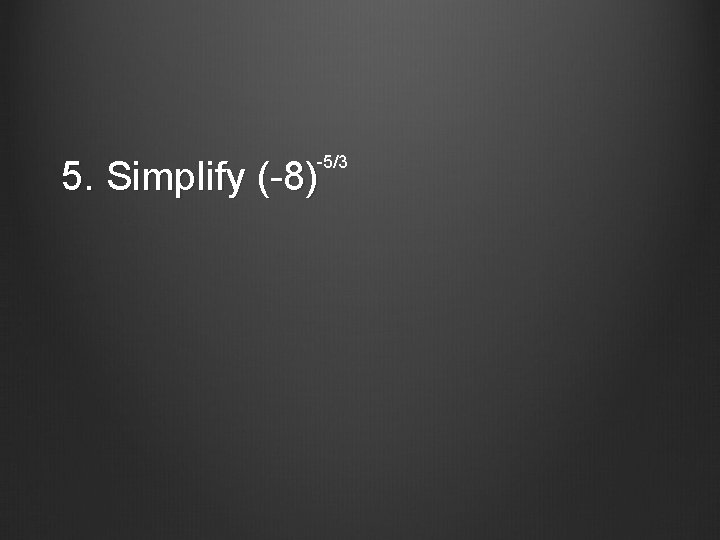-5/3 5. Simplify (-8)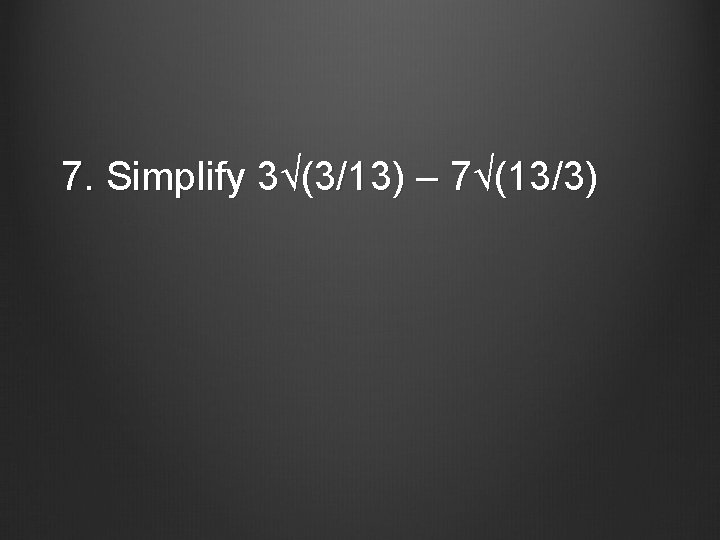7. Simplify 3√(3/13) – 7√(13/3)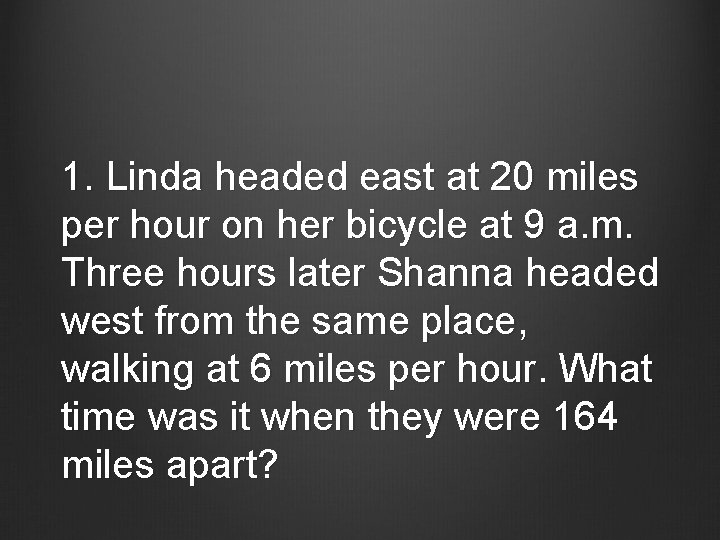1. Linda headed east at 20 miles per hour on her bicycle at 9 a. m. Three hours later Shanna headed west from the same place, walking at 6 miles per hour. What time was it when they were 164 miles apart?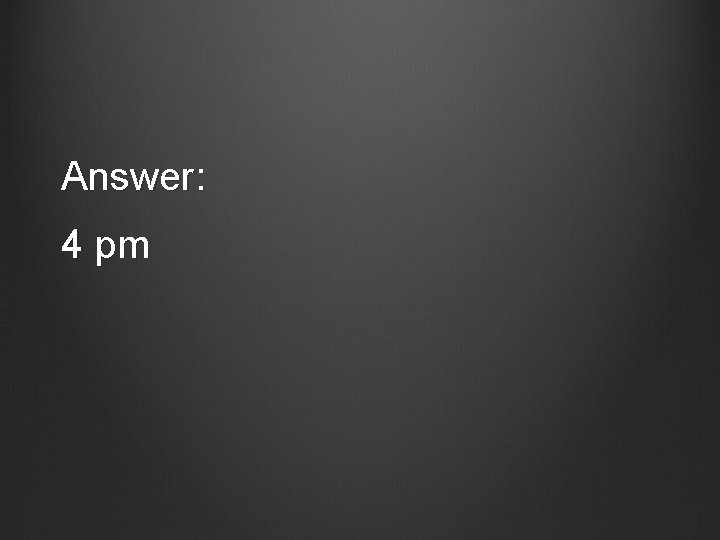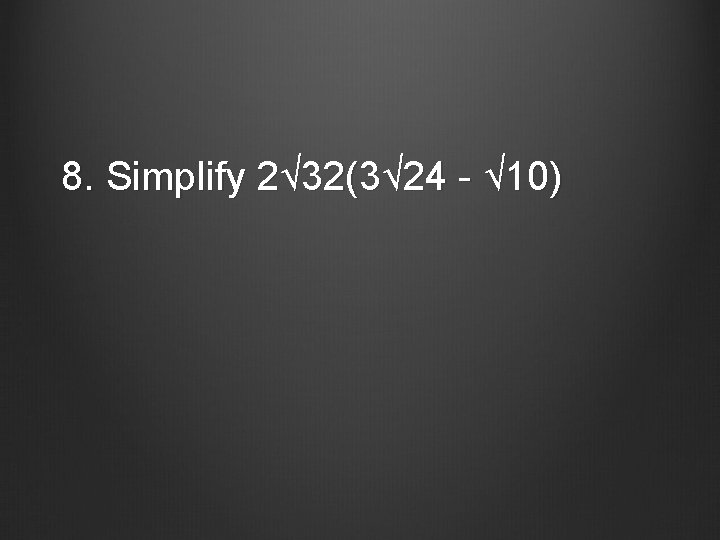8. Simplify 2√ 32(3√ 24 - √ 10)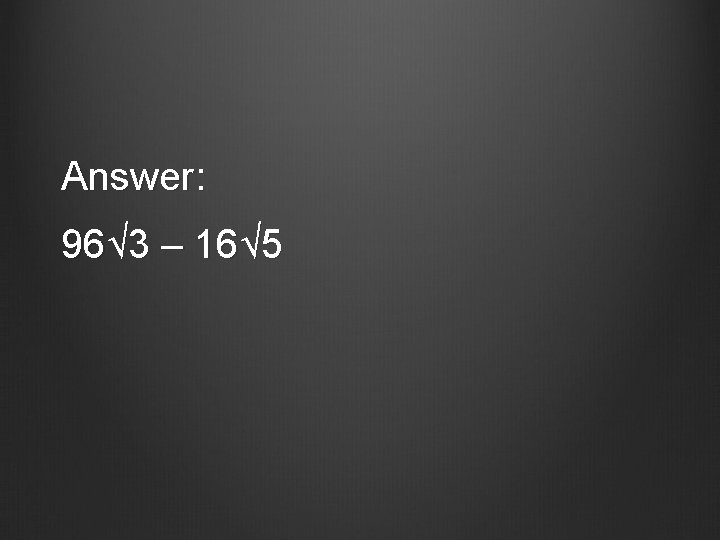Answer: 96√ 3 – 16√ 5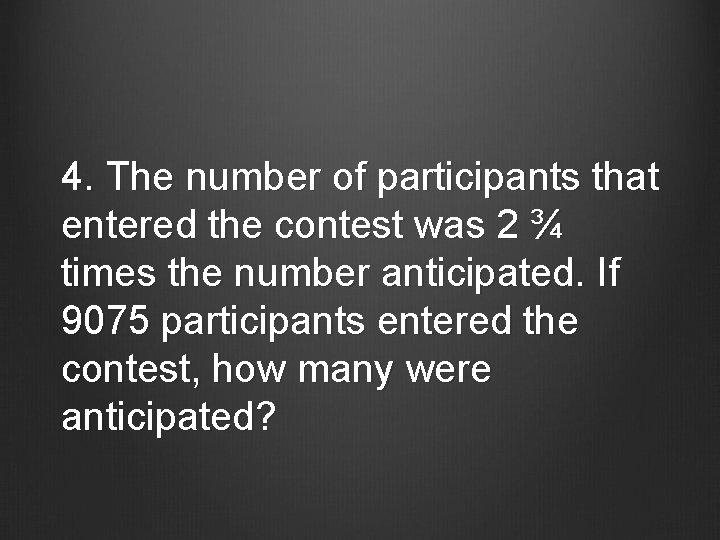4. The number of participants that entered the contest was 2 ¾ times the number anticipated. If 9075 participants entered the contest, how many were anticipated?12. Simplify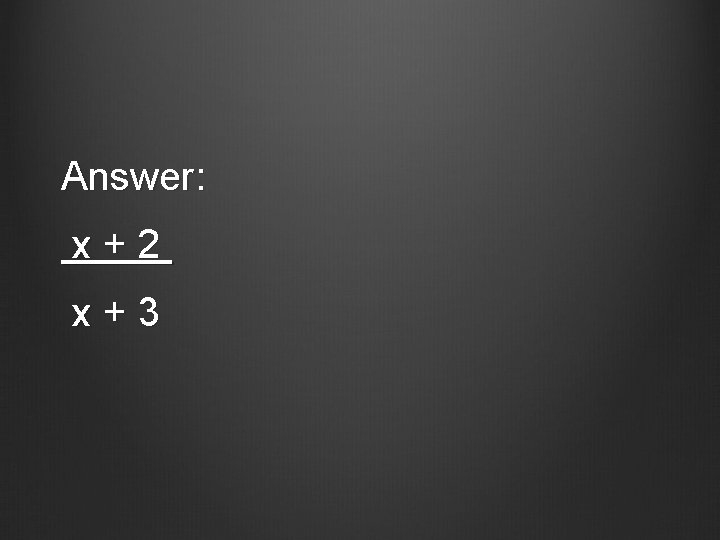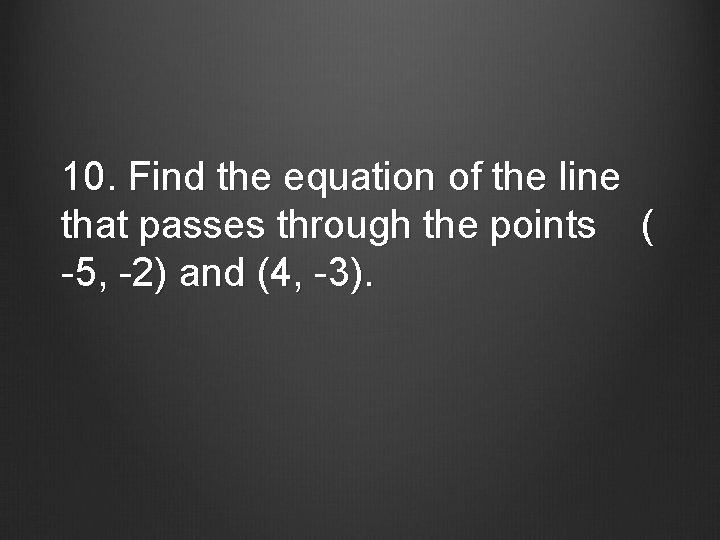10. Find the equation of the line that passes through the points ( -5, -2) and (4, -3).Answer: y = -1/9 x – 23/911. Simplify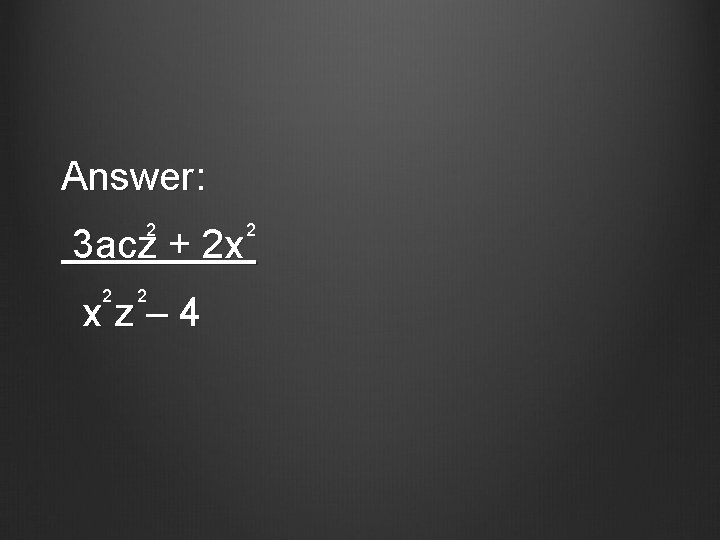Answer: 2 2 3 acz + 2 x 2 2 xz– 415. MultiplyAnswer: -1 5 m – 10 t -113. Simplify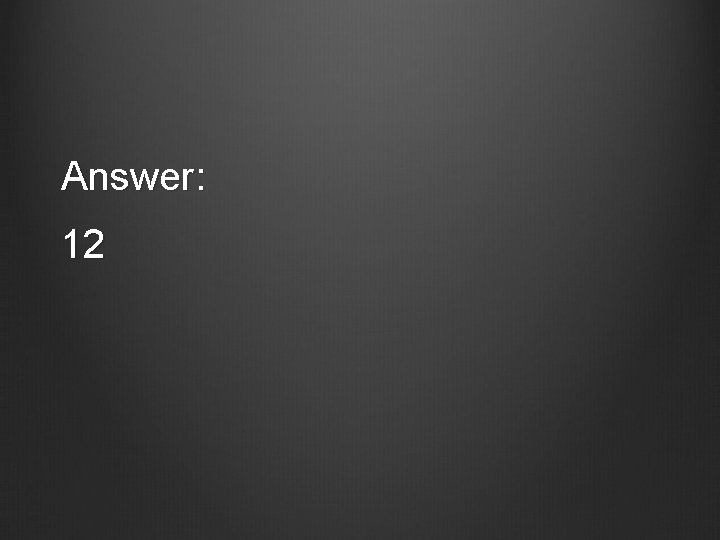Answer: 2 -3 x + 10 x + 5 (x + 2)(x – 3)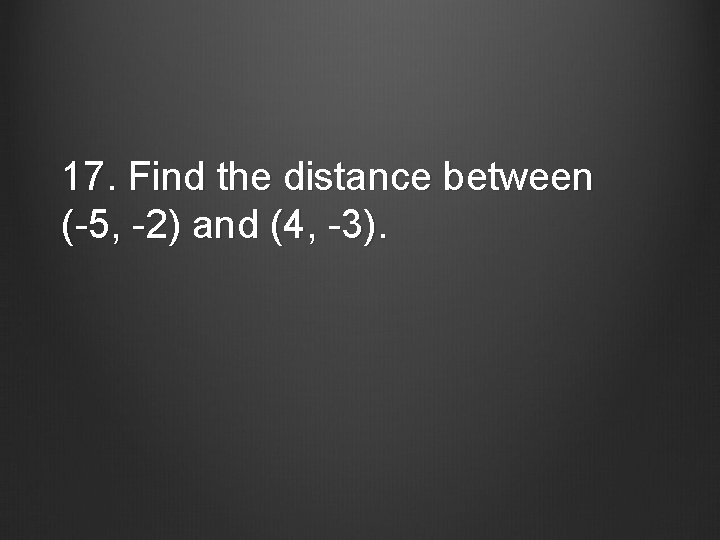17. Find the distance between (-5, -2) and (4, -3).20. Solve19. Solve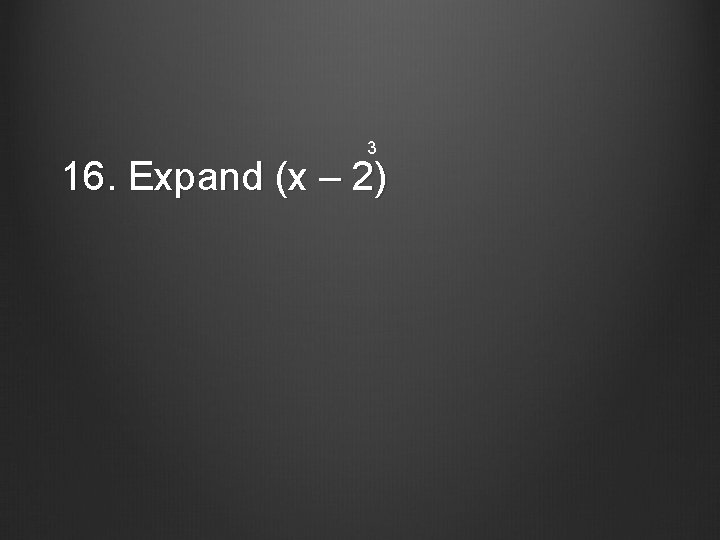3 16. Expand (x – 2)Answer: x – 6 x + 12 x – 8 3 218. Solve